# JP4848609B2 - Initial magnetic pole estimation device for AC synchronous motor - Google Patents

## Info

Publication number
JP4848609B2
JP4848609B2 JP2001294044A JP2001294044A JP4848609B2 JP 4848609 B2 JP4848609 B2 JP 4848609B2 JP 2001294044 A JP2001294044 A JP 2001294044A JP 2001294044 A JP2001294044 A JP 2001294044A JP 4848609 B2 JP4848609 B2 JP 4848609B2
Authority
JP
Japan
Prior art keywords
command
speed
magnetic pole
current
torque
Prior art date
Legal status (The legal status is an assumption and is not a legal conclusion. Google has not performed a legal analysis and makes no representation as to the accuracy of the status listed.)
Active
Application number
JP2001294044A
Other languages
Japanese (ja)
Other versions
JP2003111477A (en
Inventor

Original Assignee

Priority date (The priority date is an assumption and is not a legal conclusion. Google has not performed a legal analysis and makes no representation as to the accuracy of the date listed.)
Filing date
Publication date
Application filed by 株式会社安川電機 filed Critical 株式会社安川電機
Priority to JP2001294044A priority Critical patent/JP4848609B2/en
Publication of JP2003111477A publication Critical patent/JP2003111477A/en
Application granted granted Critical
Publication of JP4848609B2 publication Critical patent/JP4848609B2/en
Active legal-status Critical Current
Anticipated expiration legal-status Critical

• 230000001360 synchronised Effects 0.000 title claims description 38
• 244000171263 Ribes grossularia Species 0.000 claims description 141
• 281000083854 First Command Financial Planning companies 0.000 claims description 20
• 238000000034 methods Methods 0.000 description 26
• 230000029777 axis specification Effects 0.000 description 21
• 238000010586 diagrams Methods 0.000 description 18
• 230000001133 acceleration Effects 0.000 description 8
• 238000006243 chemical reactions Methods 0.000 description 7
• 230000005055 memory storage Effects 0.000 description 4
• 235000020127 ayran Nutrition 0.000 description 3
• 239000000969 carriers Substances 0.000 description 3
• 230000000694 effects Effects 0.000 description 3
• 239000001267 polyvinylpyrrolidone Substances 0.000 description 3
• 239000004173 sunset yellow FCF Substances 0.000 description 3
• 239000004374 Basic methacrylate copolymer Substances 0.000 description 2
• 239000004230 Fast Yellow AB Substances 0.000 description 2
• 239000004367 Lipases Substances 0.000 description 2
• 239000004365 Proteases Substances 0.000 description 2
• 239000004234 Yellow 2G Substances 0.000 description 2
• 230000004907 flux Effects 0.000 description 2
• 239000004149 tartrazine Substances 0.000 description 2
• 239000004229 Alkannin Substances 0.000 description 1
• 239000004366 Glucose oxidase Substances 0.000 description 1
• 239000004235 Orange GGN Substances 0.000 description 1
• 239000004372 Polyvinyl alcohol Substances 0.000 description 1
• 239000004373 Pullulan Substances 0.000 description 1
• 239000004231 Riboflavin-5-Sodium Phosphate Substances 0.000 description 1
• 230000002159 abnormal effects Effects 0.000 description 1
• 239000004676 acrylonitrile butadiene styrene Substances 0.000 description 1
• 230000000875 corresponding Effects 0.000 description 1
• 239000001573 invertase Substances 0.000 description 1
• 230000000737 periodic Effects 0.000 description 1
• 239000001253 polyvinylpolypyrrolidone Substances 0.000 description 1
• 239000004172 quinoline yellow Substances 0.000 description 1
• 239000002151 riboflavin Substances 0.000 description 1
• 230000003068 static Effects 0.000 description 1
• 230000001629 suppression Effects 0.000 description 1

## Images

•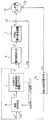•••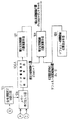•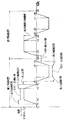•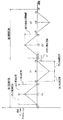•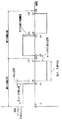•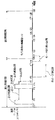••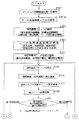•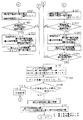••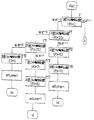••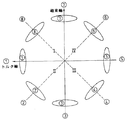•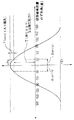•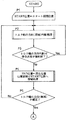•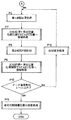## Description


BACKGROUND OF THE INVENTION
The present invention relates to performing initial magnetic pole estimation of an AC synchronous motor without using a magnetic pole sensor (pole sensor) in a permanent magnet type AC synchronous motor including a linear machine and a rotating machine.

[Prior art]
Information on the initial magnetic pole position detected from the magnetic pole detector when starting the AC synchronous motor is required, and the AC synchronous motor moves as commanded based on the information on the correct detected initial magnetic pole position. If the information on the detected initial magnetic pole position deviates ± 90 degrees from the magnetic pole position of the AC synchronous motor, no torque is generated and the AC synchronous motor does not move. Problems such as reverse running occur. For this reason, accurate detection initial magnetic pole position information is important in an AC synchronous motor, and various methods for estimating the initial magnetic pole of an AC synchronous motor have been devised in order to obtain accurate detection initial magnetic pole position information. The relationship between the deviation angle of the initial magnetic pole position and the generated torque (hereinafter, the thrust of the linear motor is also shown as torque) is shown in Expression (1) and FIG.
T = Tm × cos (θ error) (1)
Where T is the generated torque,
Tm is the maximum value of torque,
θerror is a deviation angle of the initial magnetic pole position.
In the prior art disclosed in Japanese Patent Laid-Open No. 6-153576, a voltage corresponding to an arbitrary initial magnetic pole position angle is applied, and the initial magnetic pole position is estimated from information on the motor rotation direction and motor rotation speed at that time. This is an initial magnetic pole estimation method in which the correct initial magnetic pole position is searched by repeating this process.

However, the prior art is a trial and error iterative method that estimates the true value of the initial magnetic pole position of the AC synchronous motor while approaching a narrow estimated error range from a wide estimated error range of the initial magnetic pole position.
(1) Inaccurate initial magnetic pole position cannot be estimated (the estimated accuracy range of magnetic pole position is within ± 30 °),
(2) The range of movement of the motor may increase during estimation of the initial magnetic pole position,
(3) The estimated time may take a long time,
(4) Difficult to apply to loads that have disturbances such as large static friction and cogging,
Etc. became a problem.
Therefore, the prior invention is
(1) The magnetic pole position can be estimated in a short time.
(2) The range of motor movement can be set freely.
(3) The range of motor movement can be minimized.
(4) Applicable even to loads with disturbances or large cogging torque,
(5) The true value of the initial magnetic pole position can be accurately estimated,
Therefore, the performance and characteristics of the AC synchronous motor are utilized.

First, the prior invention will be briefly described with reference to the drawings.
FIG. 1 is a schematic block diagram of speed control including an initial magnetic pole estimation apparatus handled by the prior invention. In FIG. 1, the AC motor 11 is driven by the current control means 6 and the PWM power converter 7 according to the command torque. The AC synchronous motor 11 is an AC rotary motor or an AC linear motor that does not have a magnetic pole sensor (pole sensor).
The current detection means 12 detects the current Ifb of the AC motor 11, and the electrical angle detection means (encoder) 13 detects the relative electrical angle (relative position) θfb of the AC motor.
The detection speed calculation means 14 calculates a detection speed ωfb from the detected electrical angle θfb detected by the electrical angle detection means 13.
The initial magnetic pole estimating apparatus and method 1 of the prior invention generates a command speed, the command control means 8 calculates the command torque from the information of the command speed and the detected speed, and the initial magnetic pole estimation apparatus and method of the prior invention from the information of the command torque. To obtain the estimated initial magnetic pole position of the AC synchronous motor.

FIG. 2 is a speed control block diagram based on dq current control (vector control) including the initial magnetic pole method of the AC synchronous motor according to the embodiment of the prior invention.
3 to 4 are detailed block diagrams relating to an initial magnetic pole estimation method for an AC synchronous motor according to an embodiment of the prior invention.
FIGS. 5-8 is a figure regarding the command speed pattern which has a waveform for 2 periods which concerns on embodiment of prior invention,
Figure 5 shows the trapezoidal wave command speed pattern.
Figure 6 shows the triangular wave command speed pattern.
FIG. 7 shows a rectangular wave command speed pattern.
FIG. 8 shows a command speed pattern of the zero speed wave.
However, the command speed increase / decrease pattern in the acceleration / deceleration section is arbitrary, but here, a description will be given using a primary increase / decrease function.
FIG. 9 is a diagram relating to the mode switch in the detailed block diagram of the initial magnetic pole estimation method shown in FIGS.
10 to 11 are flowcharts relating to an initial magnetic pole estimation method for an AC synchronous motor according to an embodiment of the prior invention.
The section for the command speed pattern for two periods shown in FIGS. 5 to 8 is defined as follows.
(1) t10 to t20 are first period intervals, and t20 to t30 are second period intervals. These are shown in Table 1.
[Table 1]
(2) t10 to t11 are zero speed start sections, t14 to t15 are pause sections, t18 to t21 are mode switching sections, t24 to t25 are pause sections, and t28 to t30 zero speed end sections. Zero speed start section, rest section, mode switching section, and zero speed end section), and these sections are also called rest sections. These are shown in Table 2.
[Table 2]
(3) t11 to t12, t13 to t14, t15 to t16, t17 to t18, t21 to t22, t23 to t24, t25 to t26, t27 to t28 are acceleration / deceleration sections, which are shown in Table 3.
[Table 3]
(4) t12 to t13, t16 to t17, t22 to t23, and t26 to t27 are constant speed sections. These are shown in Table 4.
[Table 4]

In each section defined above, a trapezoidal basic waveform (set time I) to applied waveform (set time II to set time IV, etc.) can be considered by setting the time for the section according to the application. . These are shown in Table 5.
[Table 5]
Here, the time of 5 ms, 50 ms, and 500 ms is an arbitrary set value, the command speed amplitude value 50 is an arbitrary set value, and the unit is r / min (for a rotary motor) or mm / sec (for a linear motor).
The data acquisition speed section provided for acquiring the magnetic pole estimation data can be selected from various sections shown in Table 6 based on the command speed pattern obtained above. These are shown in Table 6.
[Table 6]
The types of magnetic pole estimation acquisition data obtained in the data acquisition speed section can be set from the following three types.
(1) First and second maximum command torque (first and second maximum command current)
(2) First and second average command torque (first and second average command current)
(3) First and second instantaneous command torque (first and second instantaneous command current)
The first command current and the second command current can be selected from the maximum command torque, the average command torque, and the instantaneous command torque by setting the magnetic pole estimation acquisition data type, and the data acquisition calculation is expressed by the following equations (2) to (7). ).
I1 max * = MAX (I1 * [k]) (2)
I2 max * = MAX (I2 * [k]) (3)
I1 ave * = Σ (I1 * [k]) / k (4)
I2 ave * = Σ (I2 * [k]) / k (5)
I1 inst * [k] = I1 * [k] (6)
I2 inst * [k] = I2 * [k] (7)
Where I1 max * and I1 max * are the first and second maximum command currents,
I1 ave * and I1 ave * are the first and second average command currents,
I1 inst * [k] and I1 inst * [k] are the first and second instantaneous command currents,
MAX is the maximum value calculation function,
k is the number of arbitrary data.

Hereinafter, the initial magnetic pole estimation method of the prior invention will be described with reference to an example based on dq current control and the following settings.
(1) Setting 1: Command speed for 2 cycles ⇒ Trapezoidal wave command speed pattern
(2) Setting 2: Data acquisition speed section ⇒ Positive constant speed section
(3) Setting 3: Acquisition data for magnetic pole estimation ⇒ Maximum command torque (maximum command current)
In the embodiment of the prior invention, the initial magnetic pole estimation method shown in FIG. 3 is performed in the speed control loop in the dq current control means of the AC synchronous motor shown in FIG.
The dq current control of the AC synchronous motor has a configuration excluding the AC synchronous motor 11 in FIG. That is, the AC synchronous motor 11 is driven by the PWM power conversion means 72 that converts the DC voltage 74 into an arbitrary AC voltage, the three-phase current of the AC synchronous motor is detected by the three-phase current detector 12, and the electrical angle detector 13 is detected. The three-phase / two-phase coordinate conversion from the three-phase detection current to the two-phase detection current is performed by the three-phase / two-phase coordinate conversion calculation means 61 using the information on the electric angle. . The detection speed calculation means 14 calculates the detection speed ω using the detected electrical angle θ. The current error calculation means 62 subtracts the two-phase detection current from the two-phase command current to calculate the current error, and the two-phase current proportional integration control unit 63 multiplies the current error by the two-phase proportional integration gain to calculate the two-phase command voltage. Then, the 2-phase / 3-phase coordinate conversion calculation means 64 using the electrical angle information performs 2-phase / 3-phase coordinate conversion from the 2-phase command voltage to the 3-phase command voltage. The PWM gate pulse generating means 71 compares the three-phase command voltage with the triangular wave carrier 72 to calculate the PWM gate pulse, and outputs these pulses to the PWM inverter 73.
As a next step, a command speed pattern generating means 101 generates a trapezoidal command speed pattern, a speed deviation calculating means 81 subtracts the detected speed from the command speed to calculate a speed deviation, and a speed gain control unit 82 calculates the speed deviation. Multiply by the speed gain to calculate the command torque (command current).
The mode section determining means 102 determines the mode section of the first period section and the second period section from the command speed of the trapezoidal wave, and the mode switch 104 performs the mode section switching operation according to the determination result.
When switching from the first period section to the second period section (operation in which the command current calculated from the speed control means becomes an input from the q-axis command current to the d-axis command current), it is always zero speed. It is provided so as to be performed in a mode switching section which is a section. The reason is that when the mode is switched in a section other than the zero speed section (for example, an acceleration / deceleration section or a constant speed section), an abnormal operation phenomenon is likely to occur due to a component (integral term) accumulated by the speed integral gain. That is, the mode is switched by the mode switch between t17 and t21. In addition, it is provided so as to clear the integral term of the speed integral gain at the moment when the mode is switched by the mode switch.
When the first period section is selected, the command torque (command current) calculated by the speed gain control unit is input to the q-axis command current, and zero is input to the d-axis command current.
The acceleration section determination means 1102 determines whether or not the command speed is a positive acceleration section, calculates a first maximum command torque (first maximum command current) from the determined command torque, and stores it in the first memory. The data is stored in the memory by means 1104.
When the second period section is selected, zero is input to the q-axis command current, and the command torque (command current) calculated by the speed gain control unit is input to the d-axis command current.
The acceleration section determination means 1202 determines whether the command speed is a positive acceleration section, calculates a second maximum command torque (second maximum command current) from the determined command torque, and stores it in the second memory. The data is stored in the memory by means 1204.
After the second period of the command speed (from t30), the first maximum command current data and the second maximum command current data stored in the memory are called from the memory by the memory calling means 106, and the called first command current data and An excessive data judgment is performed by the data deviation excess judging means 106A from the second command current data. Thereafter, the estimated initial magnetic pole position θest is calculated by the estimated initial magnetic pole calculation means 107 of the equation (8) from the information of the called first command current data and second command current data. However, the first and second command current data (I1 data *, I2 data *) when stored in the memory are the same as the called first and second command current data (I1 data * call, I2 data * call). Value.
(1) Memory routine I: I1 data * → Memory → I2 data * call
(2) Memory routine II: I2 data * → Memory → I2 data * call
Where θest is the estimated initial magnetic pole position,
FNC is an arbitrary function,
I1 data * is the first command current data (first command torque data),
I2 data * is second command current data (second command torque data).
Finally, in addition to the default initial magnetic pole position θ0 set by the default initial magnetic pole position setting means 108 by the corrected initial magnetic pole position calculating means 109 using the equation (9), the corrected initial magnetic pole position θcomp Perform the operation.
θcomp = θ0 + θest (9)
Where θcomp is the corrected initial magnetic pole position,
θ0 is the default initial magnetic pole position (arbitrary value).
When the first command current data and the second command current data used for the calculation of the estimated initial magnetic pole position are the maximum command current, the average command current, and the instantaneous command current, the estimated initial magnetic pole position is expressed by the following equations (10) to (11). θest (θest max, θest ave, θest inst) is obtained.
θest max = tan -1 (I1 max * / I2 max *) (10)
θest ave = tan -1 (I1 ave * / I2 ave *) (11)
θest inst = Σ (tan -1 (I1 inst * [k] / I2 inst * [k])) / k (12)

FIG. 12 is a flowchart for determining the region of the torque axis or the magnetic flux axis according to the embodiment of the prior invention.
FIG. 13 is a flowchart related to the “torque axis direction determination subroutine” of S108 in the flowchart of FIG.
FIG. 14 is a flowchart following the portion (A) of the flowchart of FIG.
FIG. 15 is a diagram illustrating each direction by dividing 360 degrees according to the embodiment of the prior invention into 8 parts.
Including the process for determining the direction of the torque axis of the AC synchronous motor, the procedure for the above-described initial magnetic pole estimation calculation means is as follows.
First, the direction (region) of the torque shaft is determined based on the flowchart of FIG. 12 as follows.

<Main routine for determining the direction (region) of the torque shaft>
S100: The default initial magnetic pole position (currently set initial magnetic pole position) is set to zero. Also, the safe # area determination flag is set to 0. Proceed to S101.
S101: The first period of the waveform generated by the command speed waveform generation means is input as an axis determination command speed pattern. Proceed to S102.
S102: The speed deviation is calculated by subtracting the detected speed from the axis judgment command speed by the speed deviation calculating means. The process proceeds to S103.
S103: The speed proportional integral control unit calculates the command torque (command current) by multiplying the speed deviation by the speed proportional integral gain. However, at the moment when the direction of the torque axis is switched, the integral term of the speed integral gain is cleared. The process proceeds to S104.
S104: Command torque (command current) is input to the q-axis command current, and zero is input to the d-axis command current. The process proceeds to S105.
S105: If the result of the speed deviation excess determination means is “speed deviation ≧ speed deviation limit level”, the process proceeds to S107. Otherwise, the process proceeds to S106.
S106: If the command speed is completed, the process proceeds to S108. Otherwise, the process proceeds to S101.
S107: 1 is assigned to sp # err. Proceed to S108.
S108: The direction in which the command torque (command current) is supplied is determined in the “torque axis direction determination subroutine”. Proceed to S109.
S109: If the safe # area determination flag is 0, the process proceeds to S110. If 1, proceed to S111.
S110: Re-enter the command speed from the beginning. Proceed to S101.
S111: Proceed to Step 1 of "Initial magnetic pole estimation routine".
<Subroutine for determining the direction of the torque shaft>
F1: It is assumed that the torque axis of the AC synchronous motor is in the direction (1) in FIG. 15 (θ0 is set to 0 degree). If OK, go to F11. If NG, proceed to F12.
F10: It is assumed that there is a torque axis in the direction (4) in FIG. 15 (θ0 is set to 135 degrees). If OK, go to F101. If NG, proceed to F102.
F11: It is assumed that there is a torque axis in the direction of (8) in FIG. 15 (θ0 is set to 315 degrees). If OK, go to F111. If NG, proceed to F112.
F12: Immediately set the torque axis determination command speed to 0 and do nothing until the next axis determination command speed. Proceed to F10.
F100: It is assumed that there is a torque axis in the direction (5) in FIG. 15 (θ0 is set to 180 degrees). If OK, go to F1001. If NG, proceed to F1002.
F101: It is assumed that there is a torque axis in the direction of (3) in FIG. 15 (θ0 is set to 90 degrees). If OK, the process proceeds to F1011. If NG, proceed to F1012.
F102: Immediately set the axis determination command speed to 0 and assume that there is a torque axis in the direction of (6) in FIG. 15 (set θ0 to 225 degrees) until the next axis determination command speed . When the next axis determination command speed comes, the process proceeds to F100.
F110: Assuming that the torque axis is in the direction (3) in FIG. 15 (θ0 is set to 90 degrees), and the safe # area determination flag is set to 1.
F111: It is assumed that there is a torque axis in the direction of (7) in FIG. 15 (θ0 is set to 270 degrees). If OK, go to F1111. If NG, proceed to F1112.
F112: Immediately set the axis determination command speed to 0, and assume that there is a torque axis in the direction of (2) in FIG. 15 (set θ0 to 45 degrees) until the next axis determination command speed . When the next axis determination command speed comes, the process proceeds to F110.
F1000: Assume that there is a torque axis in the direction of (8) in FIG. 15 (set θ0 to 315 degrees), and set the safe # area determination flag to 1.
F1001: It is assumed that there is a torque axis in the direction of (7) in FIG. 15 (θ0 is set to 270 degrees), and the safe # area determination flag is set to 1.
F1002: Immediately set the axis determination command speed to 0 and assume that there is a torque axis in the direction of (7) in FIG. 15 until the next axis determination command speed (set θ0 to 270 degrees). . When the next axis determination command speed comes, the process proceeds to F1000.
F1010: Assuming that there is a torque axis in the direction of (6) in FIG. 15 (θ0 is set to 225 degrees), and the safe # area determination flag is set to 1.
F1011: It is assumed that there is a torque axis in the direction (2) in FIG. 15 (θ0 is set to 45 degrees). If OK, the process proceeds to F10111. If NG, proceed to F10112.
F1012: Immediately set the axis determination command speed to 0, and assume that there is a torque axis in the direction of (5) in FIG. 15 until the next axis determination command speed (set θ0 to 180 degrees). . When the next axis determination command speed comes, the process proceeds to F1010.
F1110: It is assumed that there is a torque axis in the direction of (2) in FIG. 15 (θ0 is set to 45 degrees), and the safe # area determination flag is set to 1.
F1111: In FIG. 15, it is assumed that there is a torque axis in the direction of (1) (θ0 is set to 0 degree), and the safe # area determination flag is set to 1.
F1112: Immediately set the axis determination command speed to 0, and assume that there is a torque axis in the direction of (1) in FIG. 15 until the next axis determination command speed (sets θ0 to 0 degrees) . When the next axis determination command speed comes, the process proceeds to F1110.
F10110: Assuming that there is a torque axis in the direction of (5) in FIG. 15 (θ0 is set to 180 degrees), and the safe # area determination flag is set to 1.
F10111: It is assumed that there is a torque axis in the direction of (4) in FIG. 15 (θ0 is set to 135 degrees), and the safe # area determination flag is set to 1.
F10112: Immediately set the axis determination command speed to 0, and assume that there is a torque axis in the direction of (4) in FIG. 15 until the next axis determination command speed (set θ0 to 135 degrees). . When the next axis determination command speed comes, the process proceeds to F10110.

<Initial magnetic pole estimation routine>
Step 1: The default initial magnetic pole position θ0 is set as an arbitrary angle (E101).
Step 1A: A data deviation limit level is set (E101A).
-Step 2: The command speed pattern generation means sets the command speed pattern (command speed amplitude value, acceleration section time, constant speed section time, pause section time, mode switching section time) and is optimal for the application field to be applied Command speed patterns (trapezoidal wave, triangle, rectangular wave, zero speed wave, sine wave) are generated (E102, E105).
Step 3: Based on the command speed pattern obtained in E102, the data acquisition speed section (see Table 2) and the magnetic pole estimation acquisition data (maximum command current, average command current, and instantaneous) obtained in the data acquisition speed section (Command current) is set (E103, E104).
Step 4: The speed deviation is calculated by subtracting the detected speed from the command speed (E106).
Step 5: Multiply the speed deviation by a speed gain (any one of speed proportional gain, speed proportional integral gain, and speed integral gain) to calculate a command torque (command current) (E107).
Step 6: The mode interval determination means performs a determination process of the mode interval (first period interval and second period interval) from the command speed, and the mode switching operation is performed from the first period interval to the second period interval according to the result. (E109).
At the moment when the mode is switched by the mode switch, the integral term processing of the velocity integral gain (clearing, holding, etc. of the integral term) is performed by the velocity integral processing means (E108).
Step 7: The operations from Step 8A to Step 8C (E1101 to E1104) are performed in the first period section obtained in E109, and Steps 9A to 9C (E1201 to E1201) are performed in the second period section obtained in E107. The operation up to E1205) is performed.
Step 8A: The command torque (command current) calculated in step 5 is input to the q-axis command current, and zero is input to the d-axis command current (E1101).
Step 8B: It is determined whether or not the command speed is the data acquisition speed section, and the first command current data (first command torque data) is calculated from the command torque in the determined section (E1102, E1103).
Step 8C: The first command current data is stored in the memory by the first memory storage means (E1104).
Step 9A: Zero is input to the q-axis command current, and the command torque (command current) calculated in step 5 is input to the d-axis command current (E1201).
Step 9B: It is determined whether or not the command speed is a data acquisition speed section, and second command current data (second command torque data) is calculated from the command torque in the determined section (E1202, E1203).
Step 9C: The second command current data is stored in the memory by the second memory storage means (E1204).
Step 10: After the end of the second period of the command speed (E1205), the first and second command current data are called from the memory (E110).
Step 11: The difference between the first command current data and the second command current data called in E110 is calculated as a data deviation (E110A).
Step 12: Compare the data deviation with the data deviation restriction level, and if the result is “data deviation ≧ data deviation restriction level”, change to a predetermined phase, and then perform the processing operation again from E105. (E110B, E110C).
Step 12A: The data deviation is compared with the data deviation limit level, and if the result is “data deviation <data deviation limit level”, the first command current data and the second command current data called in E110 are used. The estimated initial magnetic pole position is calculated by the estimated initial magnetic pole calculating means using the equation (8) (E111).
Step 13: A corrected initial magnetic pole position θcomp is calculated by adding the estimated initial magnetic pole position θest to the default initial magnetic pole position θ0 by the corrected initial magnetic pole position calculating means using equation (9) (E112).
As described above, according to the prior invention, 1) accurate initial magnetic pole position estimation can be performed in a short estimation time, 2) torque loss can be suppressed initially and maximum torque can be output, and 3) range of motor movement. There is an effect that can be minimized.

[Problems to be solved by the invention]
However, in the prior invention, the command speed pattern (triangular wave, rectangular wave, trapezoidal wave) is set so that the start initial position immediately before the start of the section operation and the end position immediately after the start of the section operation become the same position for each first period section or second period section. However, there was a phenomenon in which the position deviation slightly occurred due to disturbances such as friction and cogging when the motor was actually reciprocated. During estimation, there is a problem that the position of the mover (rotor) is shifted due to this position deviation, and an estimation error is likely to occur.
Therefore, the present invention
(1) By eliminating the position deviation in each periodic section process, the motion range being estimated becomes almost the same as the motion range by the set command speed,
(2) The true value of the initial magnetic pole position can be accurately estimated,
The purpose is to make use of the performance and characteristics of the AC synchronous motor.

To solve the above problem, The invention according to claim 1 is an initial magnetic pole estimation device for an AC synchronous motor having speed control means for calculating command torque (command current) based on the command speed and dq current control means, and using no magnetic pole detector. A command speed pattern generating means for generating an arbitrary command speed pattern waveform, a first command based on the q-axis current command with the command torque (the command current) set to a q-axis current command and a d-axis current set to zero. A first period section mode in which a command current is calculated and stored in a memory, the command torque (the command current) is set to a d-axis current command, a q-axis current is set to zero, and a second command current is calculated based on the d-axis current command. An initial magnetic pole estimation device for an AC synchronous motor, which includes a second period section mode for calculating and storing in a memory, and calculates an estimated initial magnetic pole detection position with tan-1 (the first command current / the second command current); Speed system Before starting the operation, the motor start initial position which is the motor stop position before the operation start is stored as the speed control start position, and the speed in the first period section mode or the second period section mode is stored. After completion of the control operation, operation is performed by position control from the motor end position, which is the motor stop position at the end of the operation, to the start position. An initial magnetic pole estimation apparatus for an AC synchronous motor.

DETAILED DESCRIPTION OF THE INVENTION
Hereinafter, embodiments of the present invention will be described with reference to FIGS. 17 and 18.
17 and 18 are flowcharts relating to an initial magnetic pole estimation method for an AC synchronous motor according to an embodiment of the present invention.
First, based on FIG. 17, an initial magnetic pole estimation process is performed in the following steps.
Step 1: The motor start initial position at the start of estimation is stored as the START position. P1
Step 2: Torque shaft direction determination processing is performed using the command speed for determining the direction of the torque shaft (first period section). P2
Step 3: If the command speed pattern is forcibly terminated during the process of Step 2, the process returns to Step 2; otherwise, the current position (END position) is returned to the START position in the position control mode. P3, P4
Step 4: If the direction of the torque axis is not fixed, go to Step 2. If it is fixed, the next step process is performed. P5
Step 5 (FIG. 18): The first magnetic pole estimation first period section is processed. P6
Step 6: Return the current position (END position) to the START position in the position control mode. P7
Step 7: The second magnetic pole estimation second period section is processed. P8
Step 8: Return the current position (END position) to the START position in the position control mode. P9
Step 9: If the difference between the first command current data and the second command current data obtained in Step 5 and Step 7 is greater than or equal to the data deviation limit level, go to Step 5 after performing the phase change process. The following step processing is performed. P10, P12
Step 10: The estimated initial magnetic pole position is calculated using the first command current data and the second command current data obtained in Step 5 and Step 7. P11
As described above, according to the embodiment of the present invention, the start initial position return section is provided immediately after the end of the first period section or the second period section, and the first period section or the second period section is In speed control, since the start initial position return section is operated by position control, 1) the operation range is almost the same as the operation range by the command speed, and 2) the true value of the initial magnetic pole position can be accurately estimated. effective.

【The invention's effect】
As described above, according to the present invention, the start initial position return section is provided immediately after the end of the first period section or the second period section.
1) The operation range is almost the same as the operation range by the command speed,
2) The ability to produce maximum torque while minimizing torque loss;
3) The true value of the initial magnetic pole position can be accurately estimated.
There is an effect.
[Brief description of the drawings]
FIG. 1 is a schematic block diagram of speed control including an initial magnetic pole estimation device handled by a prior invention.
FIG. 2 is a speed control block diagram based on dq current control (vector control) including an initial magnetic pole method of an AC synchronous motor according to an embodiment of the prior invention.
FIG. 3 is a detailed block diagram relating to an initial magnetic pole estimation method for an AC synchronous motor according to an embodiment of the prior invention.
FIG. 4 is a detailed block diagram related to an initial magnetic pole estimation method for an AC synchronous motor according to an embodiment of the prior invention.
FIG. 5 is a diagram relating to a command speed pattern (trapezoidal wave) having a command speed for two cycles according to the embodiment of the prior invention.
FIG. 6 is a diagram relating to a command speed pattern (triangular wave) having a command speed for two cycles according to the embodiment of the prior invention.
FIG. 7 is a diagram related to a command speed pattern (rectangular wave) having a command speed for two cycles according to the embodiment of the prior invention.
FIG. 8 is a diagram relating to a command speed pattern (zero speed wave) having a command speed for two cycles according to the embodiment of the prior invention.
9 is a diagram related to a dq mode switch in the detailed block diagram of the initial magnetic pole estimation method shown in FIG. 3;
FIG. 10 is a flowchart related to an initial magnetic pole estimation method for an AC synchronous motor according to an embodiment of the prior invention.
FIG. 11 is a flowchart relating to an initial magnetic pole estimation method for an AC synchronous motor according to an embodiment of the prior invention.
FIG. 12 is a flowchart for determining a region of a torque axis or a magnetic flux axis according to the embodiment of the prior invention.
13 is a flowchart relating to the “torque axis direction determination subroutine” of S108 in the flowchart of FIG. 12;
14 is a flowchart following the portion (A) in the flowchart of FIG.
FIG. 15 is a diagram showing 360 degrees according to the embodiment of the prior invention, divided into 8 parts, and representing each direction.
FIG. 16 is a diagram showing the relationship between the deviation angle of the initial magnetic pole position and the generated torque.
FIG. 17 is a first half of a flowchart relating to an initial magnetic pole estimation method for an AC synchronous motor according to an embodiment of the present invention;
18 is the latter half of the flowchart of FIG.
[Explanation of symbols]
* A subscript representing a directive
Subscript representing fb detection
dq two-phase coordinate system
abc Three-phase coordinate system
Vt carrier triangle wave voltage
DC voltage of Vdc PWM inverter
Vq *, Vd * d-axis and q-axis command voltages in two-phase coordinates
Va *, Vb *, Vc * Command voltage of a phase, b phase, c phase in 3 phase coordinates
Va, Vb, Vc The output voltage of the a-phase, b-phase, and c-phase inverters in the three-phase coordinates.
T * command torque
I * Command current
Tm, T, Tloss Maximum torque value, generated torque (thrust), torque loss
Iq *, Id * q-axis and d-axis command currents in two-phase coordinates
Ia, Ib, Ic Actual current of phase a, b and c in 3 phase coordinates
Iafb, Ibfb, Icfb A-phase, b-phase, and c-phase detected currents in three-phase coordinates
ΔIq, ΔId Current error of q-axis and d-axis in 2-phase coordinates
I1 data *, I2 data * First command current data and second command current data
I1 data * call, I2 data * call Call first command current data and call second command current data
I1 max *, I2 max * 1st maximum command current, 2nd maximum command current
I1 ave *, I2 ave * 1st average command current, 2nd average command current
I1 inst * [k], I2 inst * [k] First instantaneous command current at time k, second instantaneous command current at time k
θerror Deviation angle of initial magnetic pole position
Default initial magnetic pole position at θ0 initial setting
θest, θcomp Estimated initial magnetic pole position, corrected initial magnetic pole position
θest max, θest ave, θest inst Estimated initial magnetic pole position calculated with maximum command current, estimated initial magnetic pole position calculated with average command current, estimated initial magnetic pole position calculated with instantaneous command current
ω *, ωfb command speed and detection speed
Δω Speed deviation
MAX maximum value calculation function
FNC arbitrary function
ABS absolute value calculation function
Gau, Gbu, Gcu, Gad, Gbd, Gcd 6 gate pulses of PWM inverter
sp # err Speed deviation judgment flag
safe # area Axial direction judgment flag
1 Early magnetic pole estimation apparatus and method of the prior invention
6 Current control means
7 PWM power converter
8 Speed control means
11 AC synchronous motor (rotary motor or linear motor)
12 Three-phase AC current detector (CT)
13 Encoder
14 Detection speed calculation means
15 Correction initial magnetic pole position calculation means
61 3/2 coordinate conversion calculation means
62 Subtractor (current error calculation means)
63 Current proportional integral controller
64 2/3 coordinate conversion calculation means
71 PWM gate pulse generator
72 Triangular carrier
73 PWM inverter
74 DC power supply
81 Subtractor (speed deviation calculation means)
82 Speed gain controller
101 Command speed pattern generation means
102 Mode section judgment means
103 Speed integral gain processing means
104 Mode switch
1101 1st period section (q-axis command current ← command torque, d-axis command current ← 0)
1102 Speed section judgment means for data acquisition
1103 First command current calculation means
1104 First memory storage means
1201 2nd period section (q-axis command current ← 0, d-axis command current ← command torque)
1202 Data acquisition speed interval determination means
1203 Second command current calculation means
1204 Second memory storage means
105 Command speed end judging means
106 Memory calling means
106A Data deviation excess judgment means
107 Estimated initial magnetic pole position calculating means
108 Default initial magnetic pole position setting means
109 Correction initial magnetic pole position calculation means

## Claims (1)

1. An initial magnetic pole estimation device for an AC synchronous motor having speed control means for calculating a command torque (command current) based on a command speed and dq current control means, and using no magnetic pole detector,
Command speed pattern generating means for generating an arbitrary command speed pattern waveform;
A first period section mode in which the command torque (the command current) is a q-axis current command, a d-axis current is zero, a first command current is calculated based on the q-axis current command and stored in a memory;
A second period section mode in which the command torque (the command current) is a d-axis current command, the q-axis current is zero, a second command current is calculated based on the d-axis current command and stored in a memory;
In an initial magnetic pole estimation device for an AC synchronous motor that calculates an estimated initial magnetic pole detection position with tan-1 (the first command current / the second command current),
Before starting operation in speed control, store the motor start initial position that is the motor stop position before the start of operation as the start position of the speed control,
After the operation in the speed control in the first cycle section mode or the second cycle section mode is completed, the operation is performed by the position control from the motor end position which is the motor stop position at the time after the operation is completed to the start position. A device for estimating an initial magnetic pole of an AC synchronous motor.
JP2001294044A 2001-09-26 2001-09-26 Initial magnetic pole estimation device for AC synchronous motor Active JP4848609B2 (en)

## Priority Applications (1)

Application Number Priority Date Filing Date Title
JP2001294044A JP4848609B2 (en) 2001-09-26 2001-09-26 Initial magnetic pole estimation device for AC synchronous motor

## Applications Claiming Priority (1)

Application Number Priority Date Filing Date Title
JP2001294044A JP4848609B2 (en) 2001-09-26 2001-09-26 Initial magnetic pole estimation device for AC synchronous motor

## Publications (2)

Publication Number Publication Date
JP2003111477A JP2003111477A (en) 2003-04-11
JP4848609B2 true JP4848609B2 (en) 2011-12-28

# Family

## Family Applications (1)

Application Number Title Priority Date Filing Date
JP2001294044A Active JP4848609B2 (en) 2001-09-26 2001-09-26 Initial magnetic pole estimation device for AC synchronous motor

## Country Status (1)

JP (1) JP4848609B2 (en)

## Families Citing this family (2)

* Cited by examiner, † Cited by third party
Publication number Priority date Publication date Assignee Title
WO2007034689A1 (en) * 2005-09-26 2007-03-29 Kabushiki Kaisha Yaskawa Denki Ac synchronous motor initial magnetic pole position estimation device and its method
JP5957696B2 (en) * 2012-08-07 2016-07-27 パナソニックＩｐマネジメント株式会社 Motor drive device

## Family Cites Families (2)

* Cited by examiner, † Cited by third party
Publication number Priority date Publication date Assignee Title
JPH06153576A (en) * 1992-10-30 1994-05-31 Sharp Corp Detecting apparatus for position of magnetic pole of ac servomotor
JP4239372B2 (en) * 1999-09-17 2009-03-18 株式会社安川電機 Initial magnetic pole estimation device for AC synchronous motor

## Also Published As

Publication number Publication date
JP2003111477A (en) 2003-04-11

## Similar Documents

Publication Publication Date Title
Piippo et al. Analysis of an adaptive observer for sensorless control of interior permanent magnet synchronous motors
US8253360B2 (en) Vector controlled motor drive system implementing pulse width modulated (PWM) waveforms
JP3668870B2 (en) Synchronous motor drive system
JP4531751B2 (en) Synchronous machine controller
EP1061640B2 (en) A method and apparatus for energizing stator windings of a rotating electromagnetic machine
EP0748038B1 (en) System and method for controlling brushless permanent magnet motors
JP4067949B2 (en) Motor control device
EP2770627B1 (en) Motor control device and motor control method
EP1133050B1 (en) Method of estimating a rotor position of synchronous motor, method of controlling synchronous motor with no position sensor and a controller of synchronous motor
JP4496410B2 (en) Motor control device
EP2034605B1 (en) Electric motor driving device, and compressor driving device
Chen et al. An extended electromotive force model for sensorless control of interior permanent-magnet synchronous motors
JP5652664B2 (en) Rotating electrical machine control device
JP3972124B2 (en) Synchronous motor speed control device
US6650083B2 (en) Speed control apparatus of synchronous reluctance motor and method thereof
JP3681318B2 (en) Synchronous motor control device and vehicle using the same
DE10218728B4 (en) Engine control / regulating device
JP4063166B2 (en) Electric motor control device
US9077275B2 (en) Rotor position estimating device, electric motor control system and rotor position estimating method
JP3860031B2 (en) Synchronous motor control device and control method of synchronous motor
DE102007013575B4 (en) motor control
US8159161B2 (en) Motor control device
US8174220B2 (en) Apparatus for controlling permanent-magnet rotary electric machine
JP3813637B2 (en) AC motor control device
EP1986318B1 (en) Motor control device

## Legal Events

Date Code Title Description
RD04 Notification of resignation of power of attorney

Free format text: JAPANESE INTERMEDIATE CODE: A7424

Effective date: 20060324

RD04 Notification of resignation of power of attorney

Free format text: JAPANESE INTERMEDIATE CODE: A7424

Effective date: 20071127

A621 Written request for application examination

Free format text: JAPANESE INTERMEDIATE CODE: A621

Effective date: 20080818

A977 Report on retrieval

Free format text: JAPANESE INTERMEDIATE CODE: A971007

Effective date: 20110127

A131 Notification of reasons for refusal

Free format text: JAPANESE INTERMEDIATE CODE: A131

Effective date: 20110201

A521 Written amendment

Free format text: JAPANESE INTERMEDIATE CODE: A523

Effective date: 20110217

TRDD Decision of grant or rejection written
A01 Written decision to grant a patent or to grant a registration (utility model)

Free format text: JAPANESE INTERMEDIATE CODE: A01

Effective date: 20110920

A01 Written decision to grant a patent or to grant a registration (utility model)

Free format text: JAPANESE INTERMEDIATE CODE: A01

A61 First payment of annual fees (during grant procedure)

Free format text: JAPANESE INTERMEDIATE CODE: A61

Effective date: 20111003

R150 Certificate of patent or registration of utility model

Ref document number: 4848609

Country of ref document: JP

Free format text: JAPANESE INTERMEDIATE CODE: R150

Free format text: JAPANESE INTERMEDIATE CODE: R150

FPAY Renewal fee payment (event date is renewal date of database)

Free format text: PAYMENT UNTIL: 20141028

Year of fee payment: 3